Friday, October 22, 2021
Home > IMO > Pre RMO Previous Year 2014 Question Paper With Solutions

# Pre RMO Previous Year 2014 Question Paper With Solutions# Pre RMO Previous Year 2014 Question Paper With Solutions

In this post, you will get Pre RMO Previous Year 2014 Question Paper With Solutions.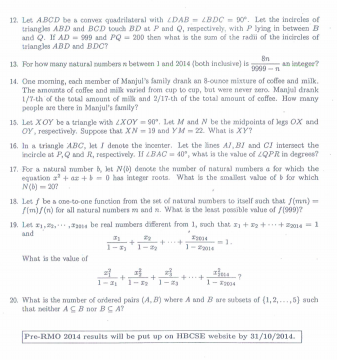Pre RMO Previous Year 2014 Question Paper Question No 1:

A natural number k is such that k2 < 2014 < (k + 1)2. What is the largest prime factor of k?

Pre RMO Previous Year 2014 Question Paper Solution of Question No 1: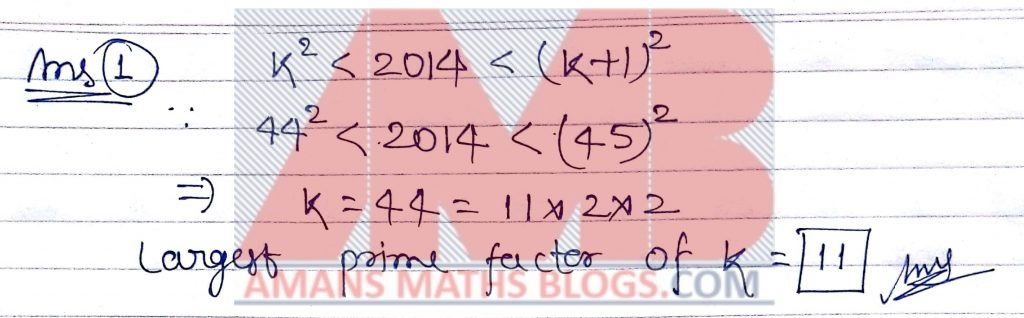Pre RMO Previous Year 2014 Question Paper Question No 2:

The first term of a sequence is 2014. Each succeeding term is the sum of the cubes of the digits of the previous term. What is the 2014th term of the sequence?

Pre RMO Previous Year 2014 Question Paper Solution of Question No 2: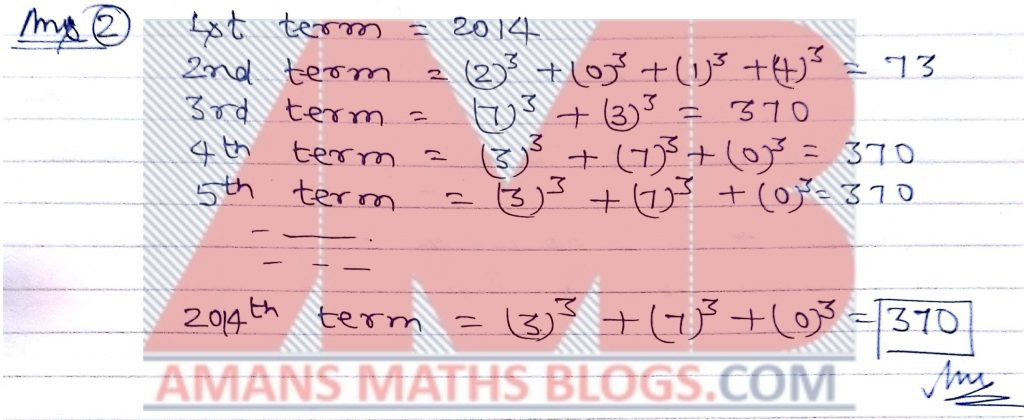Pre RMO Previous Year 2014 Question Paper Question No 3:

Let ABCD be a convex quadrilateral with perpendicular diagonals. If AB = 20, BC = 70 and CD = 90, then what is the value of DA?

Pre RMO Previous Year 2014 Question Paper Solution of Question No 3: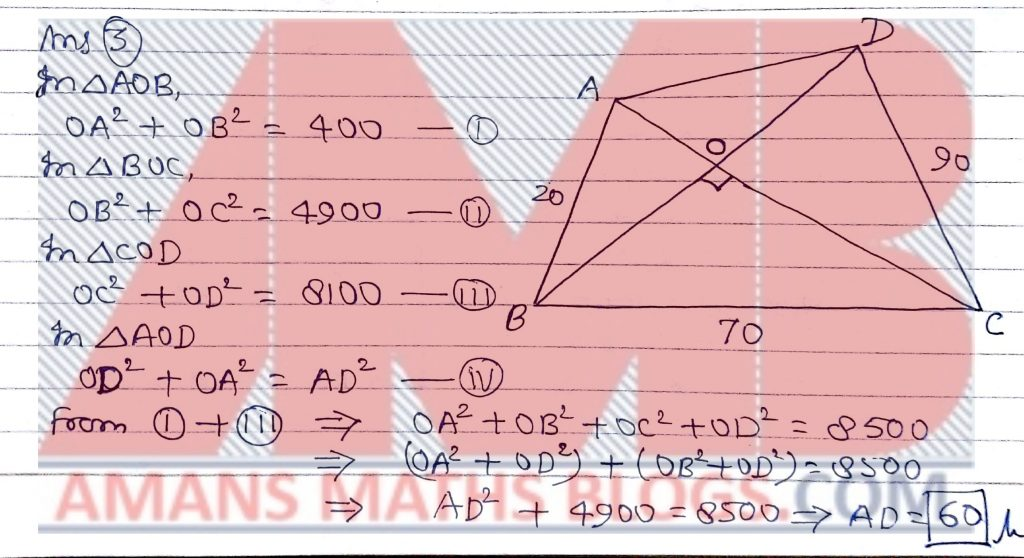Pre RMO Previous Year 2014 Question Paper Question No 4:

In a triangle with integer side lengths, one side is three times as long as a second side, and the length of the third side is 17. What is the greatest possible perimeter of the triangle?

Pre RMO Previous Year 2014 Question Paper Solution of Question No 4: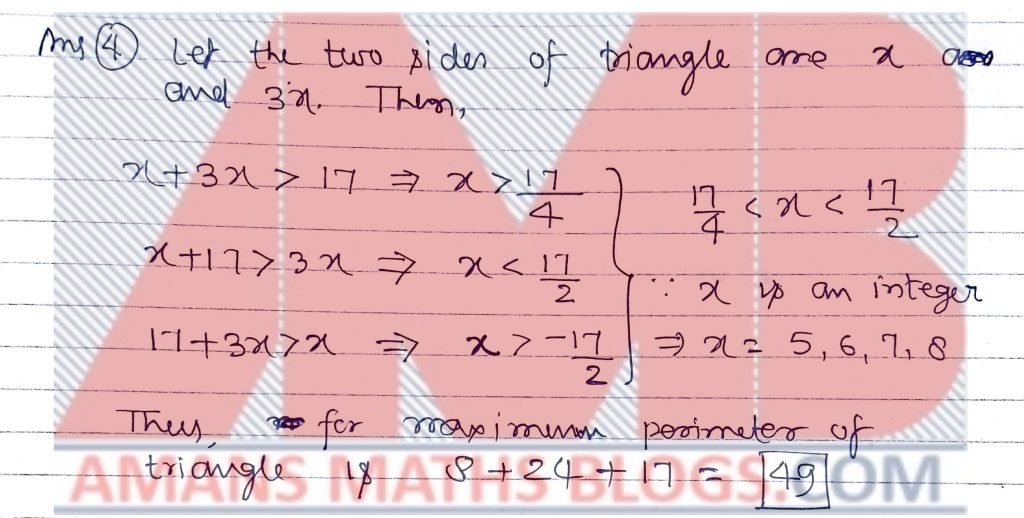Pre RMO Previous Year 2014 Question Paper Question No 5:

If real numbers a, b, c, d, e satisfy a + 1 = 2 + b = c + 3 = d + 4 = e + 5 = a + b + c + d + e + 3. What is the value of a2 + b2 + c2 + d2 + e2?

Pre RMO Previous Year 2014 Question Paper Solution of Question No 5: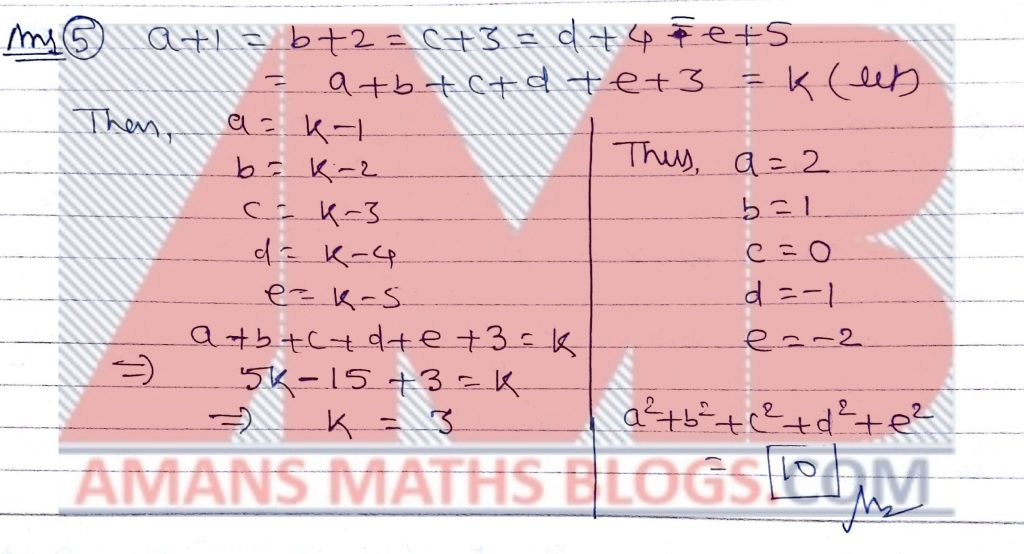Pre RMO Previous Year 2014 Question Paper Question No 6:

What is the smallest possible natural number n for which the equation x2 – nx + 2014 = 0 has integer roots?

Pre RMO Previous Year 2014 Question Paper Solution of Question No 6: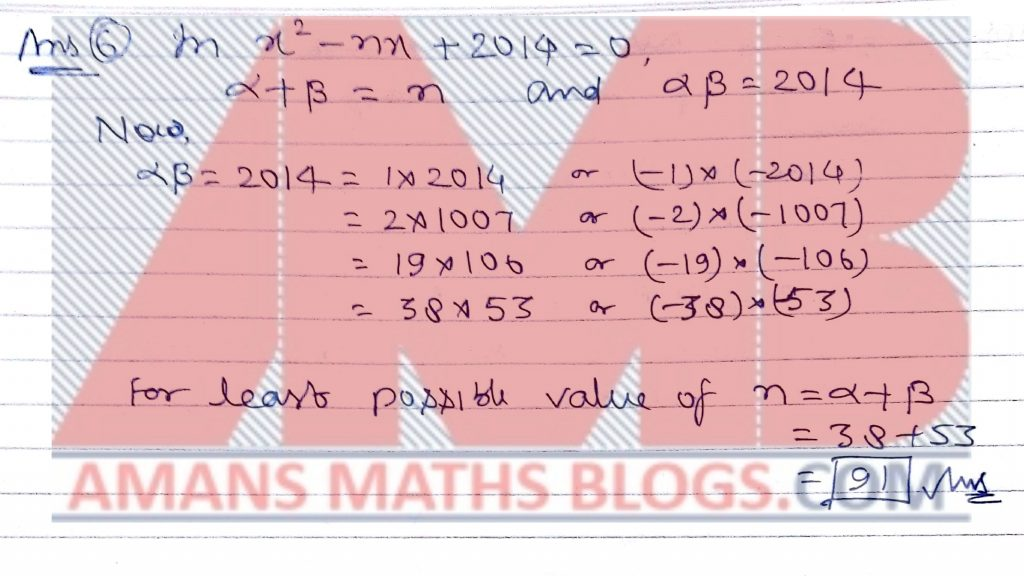Pre RMO Previous Year 2014 Question Paper Question No 7:

If, what is the value of?

Pre RMO Previous Year 2014 Question Paper Solution of Question No 7: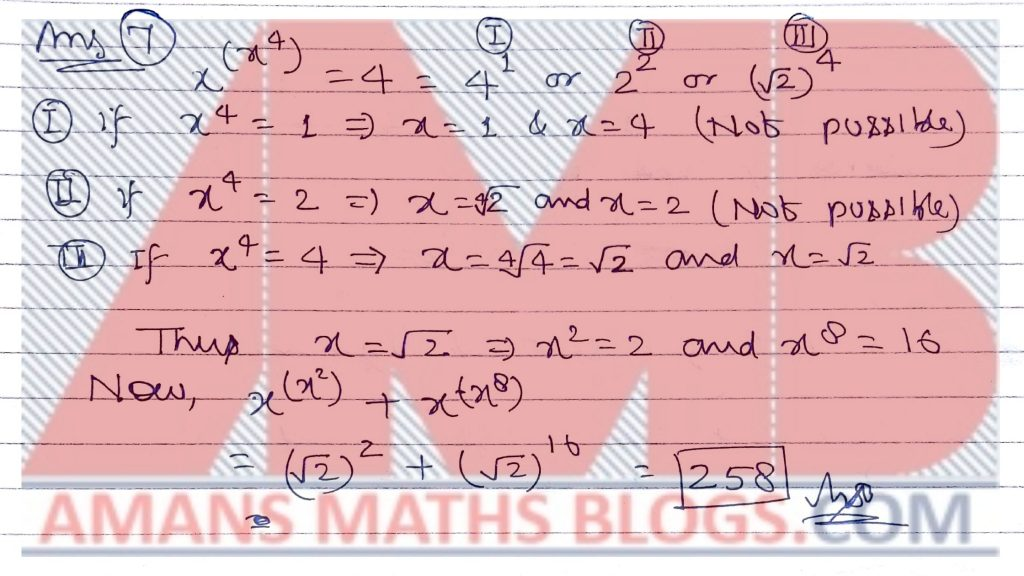Pre RMO Previous Year 2014 Question Paper Question No 8:

Let S be a set of real numbers with mean M. If the means of the sets S U {15} and S U {15,1} are M + 2 and M + 1, respectively, then how many elements does S have?

Pre RMO Previous Year 2014 Question Paper Solution of Question No 8: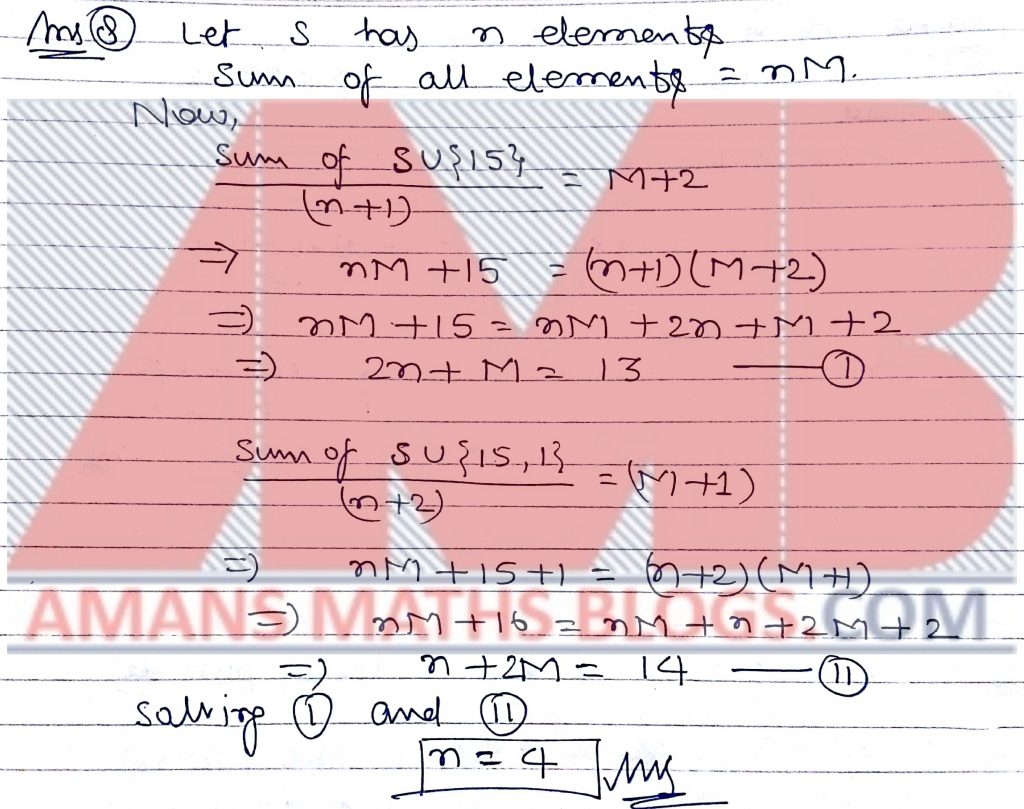Pre RMO Previous Year 2014 Question Paper Question No 9:

Natural numbers k, I, p and q are such that if a and b are roots of x2 – kx + l = 0  then a + 1/b and b + 1/a are the roots x2 – px + q = 0. What is the sum of all possible values of q?

Pre RMO Previous Year 2014 Question Paper Solution of Question No 9: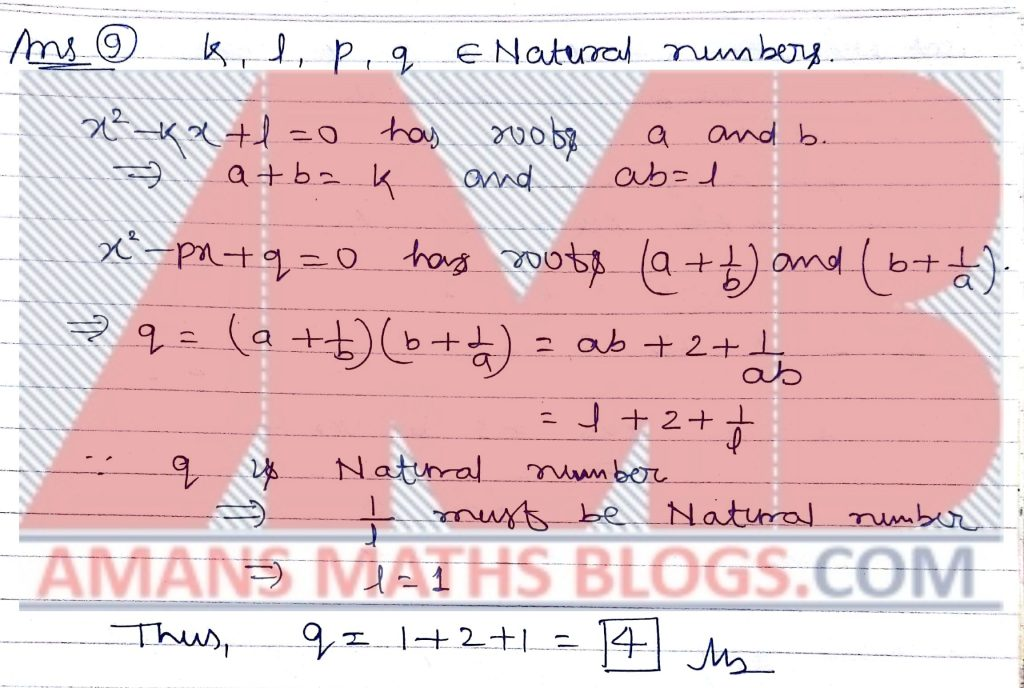Pre RMO Previous Year 2014 Question Paper Question No 10:

In a triangle ABC, X and Y are points on the segments AB and AC, respectively, such that AX : XB = 1 : 2 and AY : YC = 2 : 1. If the area of triangle AXY is 10 then what is the area of triangle ABC?

Pre RMO Previous Year 2014 Question Paper Solution of Question No 10: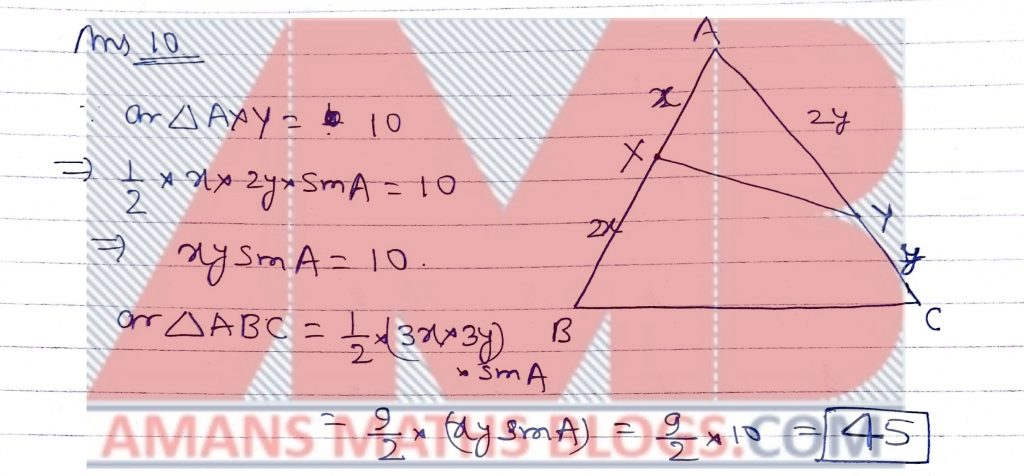Pre RMO Previous Year 2014 Question Paper Question No 11:

For natural numbers x and y, let (x, y) denote the greatest common divisor of x and y. How many pairs of natural numbers x and y with x < y satisfy the equation xy = x + y + (x, y)?

Pre RMO Previous Year 2014 Question Paper Solution of Question No 11: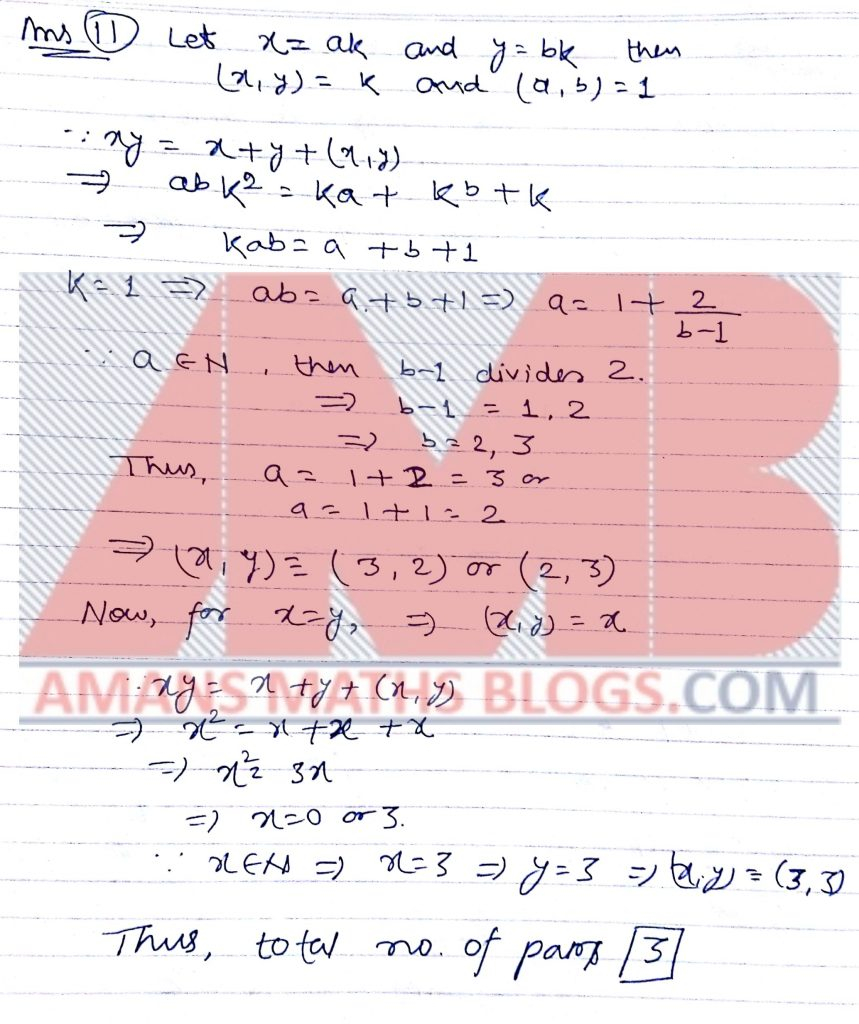Pre RMO Previous Year 2014 Question Paper Question No 12:

Let ABCD be a convex quadrilateral with angle DAB = angle BDC = 90 degrees. Let the incircles of triangles ABD and BCD touch DB at P and Q, respectively, with P lying in between B and Q. If AD = 999 and PQ = 200 then what is the sum of the radii of the incircles of triangle ABD and BDC?

Pre RMO Previous Year 2014 Question Paper Solution of Question No 12: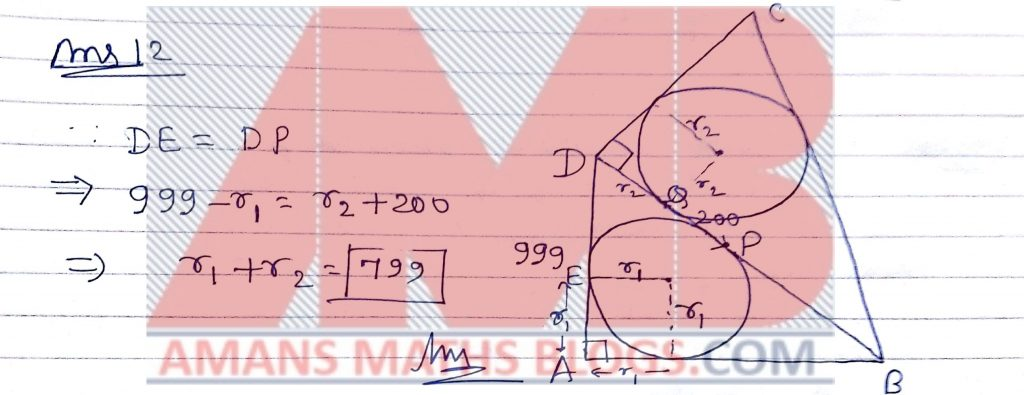Pre RMO Previous Year 2014 Question Paper Question No 13:

For how many natural numbers n between 1 and 2014 (both inclusive) isan integer?

Pre RMO Previous Year 2014 Question Paper Solution of Question No 13: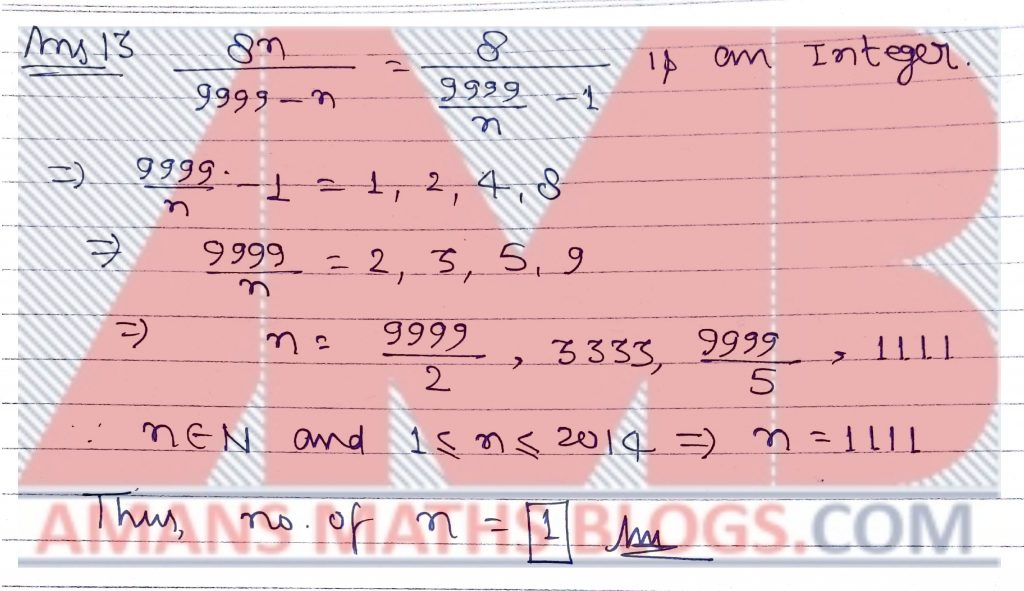Pre RMO Previous Year 2014 Question Paper Question No 14:

One morning, each member of Manjul’s family drank and 8-ounce mixture of coffee and milk. The amounts of coffee and milk varied from cup to cup, but were never zero. Manjul drank 1/7-th of the total amount of milk and 2/7-th of the total amount of coffee. How many people are there in Manjul’s family?

Pre RMO Previous Year 2014 Question Paper Solution of Question No 14: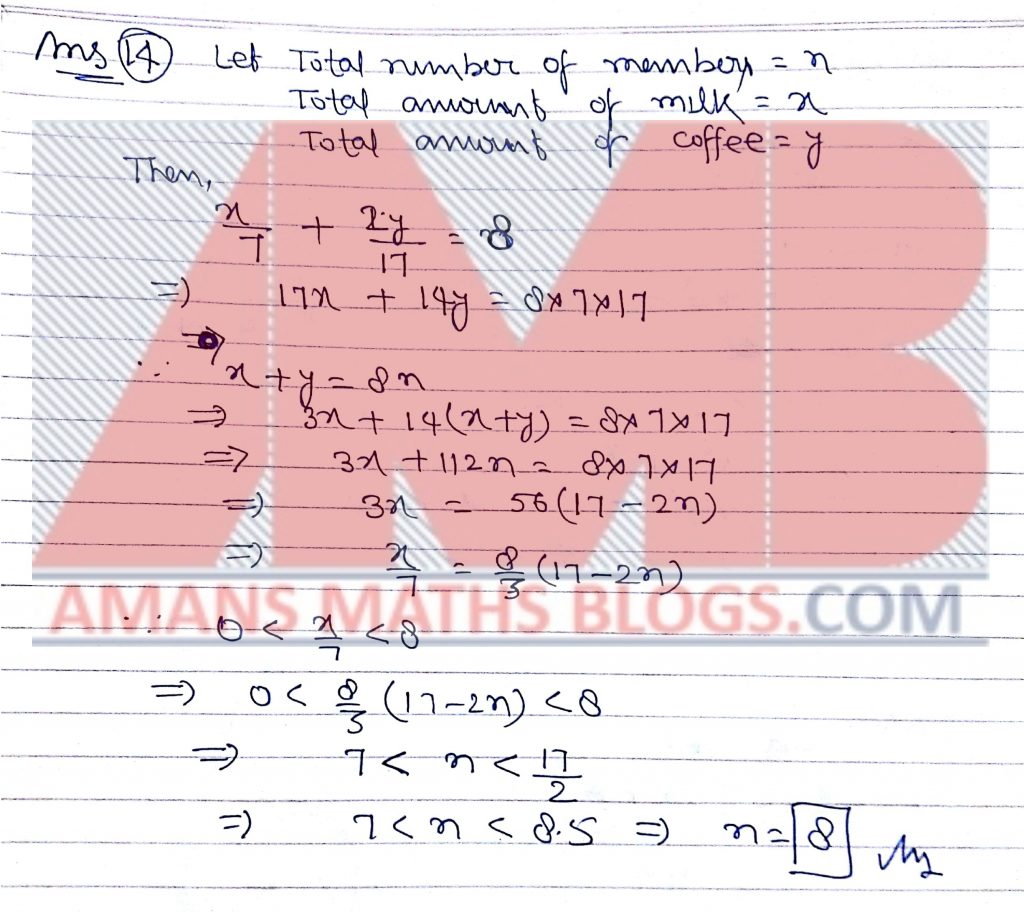Pre RMO Previous Year 2014 Question Paper Question No 15:

Let XOY be a triangle with  angle XOY = 90 degrees. Let M and N be the midpoints of legs OX and OY, respectively. Suppose that XN = 19 and YM = 22. What is XY?

Pre RMO Previous Year 2014 Question Paper with Solutions of Question No 15: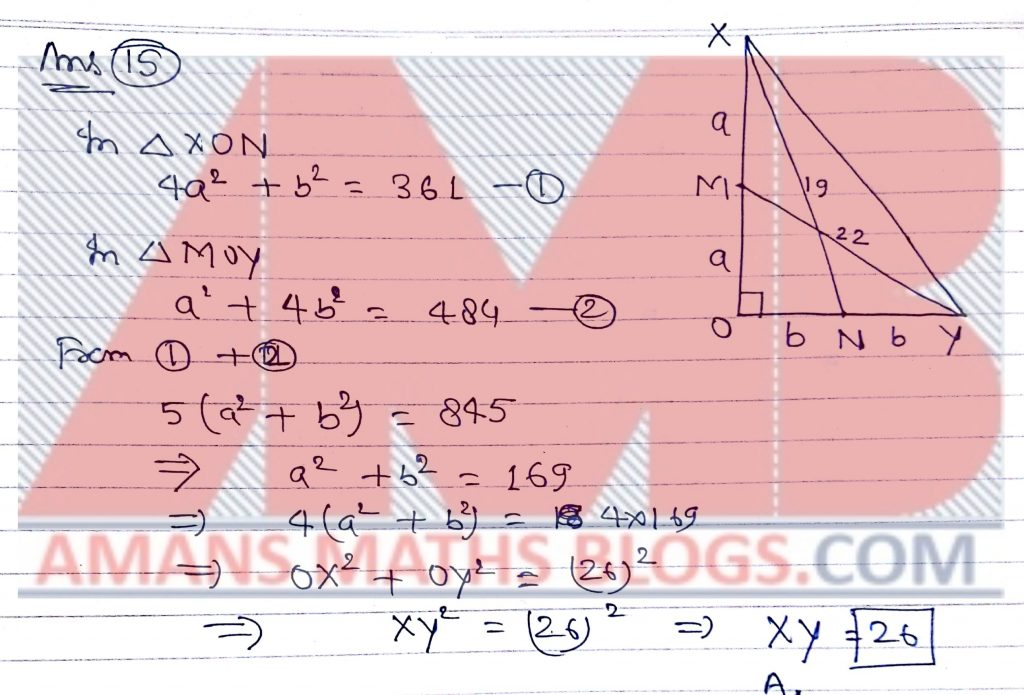Pre RMO Previous Year 2014 Question Paper Question No 16:

In a triangle ABC, let I denote the inceter. Let the lines AI, BI and CI intersect the incircle at P, Q and R, respectively. If  angle BAC = 40 degrees, what is value of angle QPR in degrees?

Pre RMO Previous Year 2014 Question Paper Solution of Question No 16: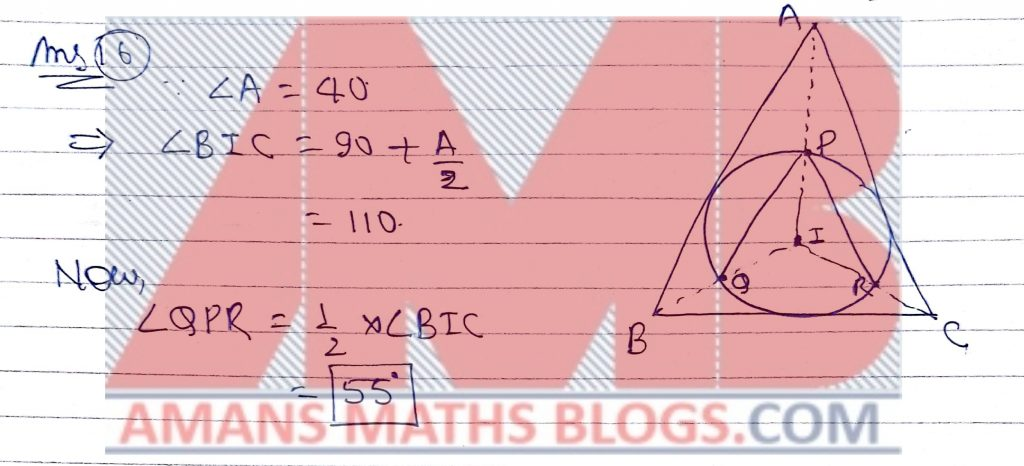Pre RMO Previous Year 2014 Question Paper Question No 17:

For a natural number b, let N(b) denote of natural numbers a for which the equation x2 + ax + b = 0 has integer roots. What is the smallest value of b for which N(b) = 20?

Pre RMO Previous Year 2014 Question Paper Solution of Question No 17:

Pre RMO Previous Year 2014 Question Paper Question No 18:

Let f be a one-to-one function from the set of natural numbers to itself such that f(mn) = f(m) f(n) for all natural numbers m and n. What is the least possible value of f(999)?

Pre RMO Previous Year 2014 Question Paper Solution of Question No 18:

Pre RMO Previous Year 2014 Question Paper Question No 19:

Let x1, x2, x3, …, x2014 be real numbers different from 1 such that x1 + x+ x+ …+ x2014 = 1 and. What is the value of?

Pre RMO Previous Year 2014 Question Paper Solution of Question No 19: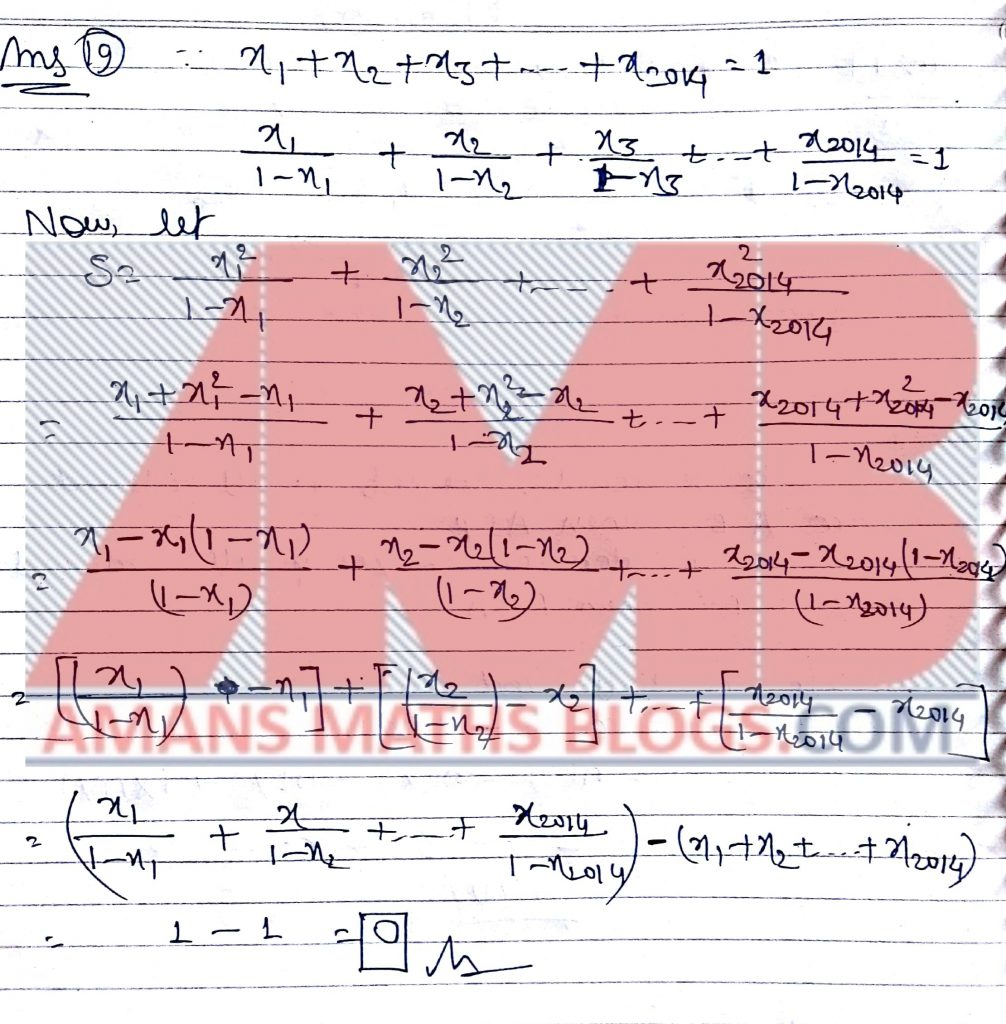Pre RMO Previous Year 2014 Question Paper Question No 20:

What is the number of ordered pairs (A, B) where A and B are subsets of {1,2,….,5) such that neither AB not BA?

Pre RMO Previous Year 2014 Question Paper Solution of Question No 20: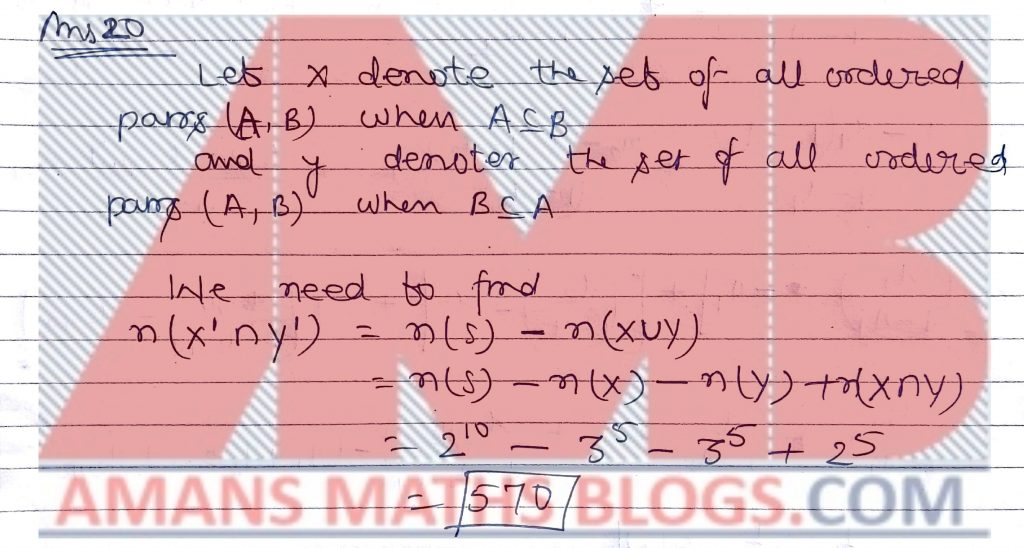Get Combined Pre RMO 2012 to 2018 Questions Paper with Solution (Click on Image)
AMBiPi
error: Content is protected !!